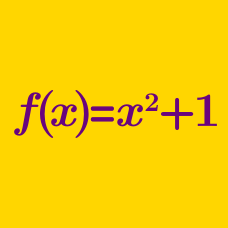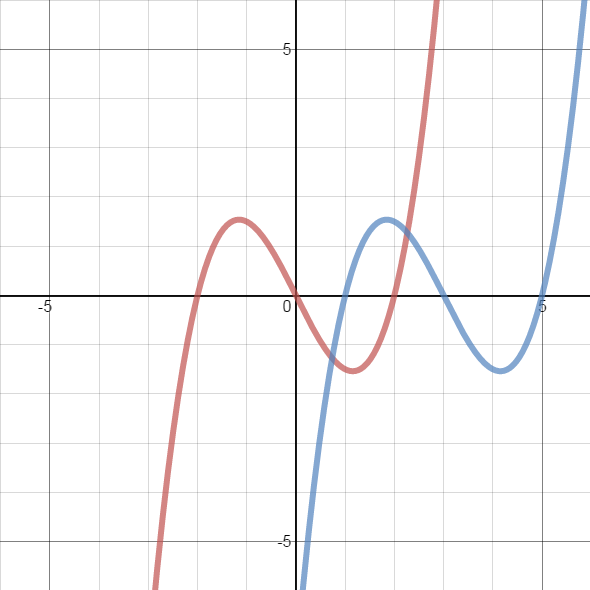Algebra

# Function Misconceptions

$f$ and $g$ are two functions such that $(f \circ g)(3)=5$

What is the value of $(g \circ f)(3)$ ?

If $f$ is a function with domain $\mathbb{R}$ and range $\mathbb{R}$, does there exist a function $g$ such that $g(x) = f^{-1}(x)$ ?In the above image, the red graph is a graph of $y=f(x)$, for some function $f$.

What transformation of $f$ will produce the blue graph in the above graph?

$f(x)$ is a function with domain $\mathbb{R}.$

$g(x)$ is a horizontal transformation of $f(x)$ such that $g(x) = f(x+k).$

$h(x)$ is a vertical transformation of $f(x)$ such that $h(x) = f(x)+k.$

If you choose $f(x)$ carefully, is it possible that $g(x)$ might equal $h(x)$ for all $x$?

$f$ is a function whose domain is $\mathbb{R}$.

$g$ is a function such that $g(x)=f^{-1}(x)$

True or false: The domain of $g$ must also be $\mathbb{R}$.

×